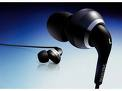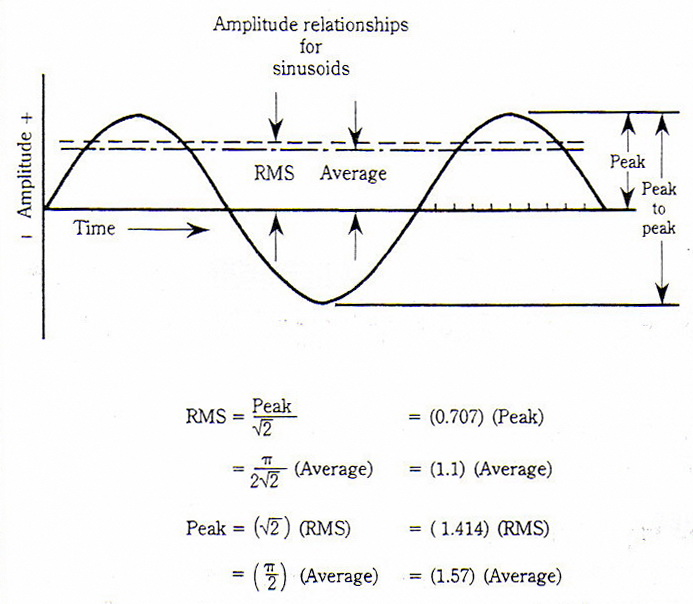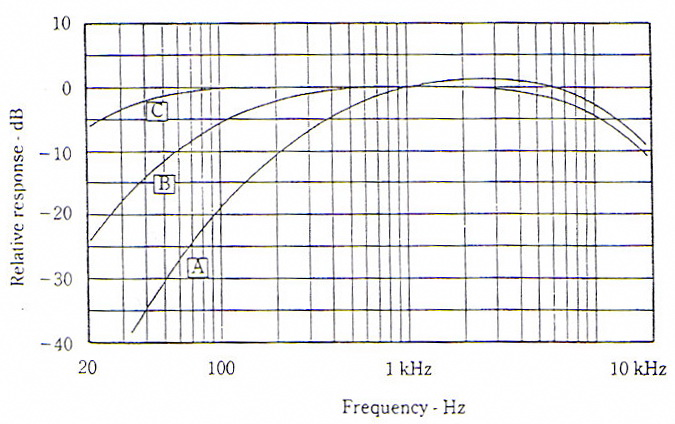►TOYSAcousticsdB – decibel   The sound pressure level, Lp, in dB’s is defined as 20 log p/po, where p is the measured value in Pa and po is a standardized reference level of 20 x 10-6 Pa – the threshold of hearing. Note here that the word level is added to sound pressure to indicate that the quantity has a certain level above the reference level and the symbol for sound pressure level is Lp.   Lp = 20 log (P/Po) dB   Po = 20 x 10-6 Pa   Sine-Wave Language   The sine wave is a specific kind of alternating signal and is described by its own set of specific terms. Viewed on an oscilloscope, the easiest value to read is the peak-to-peak value (of voltage, current, sound pressure, or whatever the sine wave represents), the meaning of which is obvious in Fig. 1. If the wave is symmetrical, the peak-to-peak value is twice the peak value.   The common ac voltmeter is, in reality, a dc instrument fitted with a rectifier that changes the alternating sine current to pulsating unidirectional current. The dc meter then responds to the average value as indicated in Fig. 1. Such meters are, however, almost universally calibrated in terms of rms (described in the next paragraph). For pure sine waves, this is quite an acceptable fiction, but for nonsinusoidal wave-shapes the reading will be in error.   An alternating current of one ampere rms (or effective) is exactly equivalent in heating power to 1 ampere of direct current as it flows through a resistance of known value. After all, alternating current can heat up a resistor or do work no matter which direction if flows, it is just a matter of evaluating it. In the right-hand positive loop of Fig. 1 the ordinates (height of lines to the curve) are read off for each marked increment of time. Then (a) each of these ordinate values is squared. (b) the squared values are added together, (c) the average is found, and (d) the square root is taken of the average (or mean). Taking the square root of this average gives the root-mean-square or rms value of the positive loop of Fig. 1. The same can be done for the negative loop (squaring a negative ordinate gives a positive value), but simply doubling the positive loop of a symmetrical wave is easier. In this way the root-mean-square or “heating power” value of any alternating or periodic waves can be determined whether the wave is for voltage, current, or sound pressure. Such computations will help you understand the meaning of rms, but fortunately reading meters is far easier. Figure 1 is a useful summary of relationships pertaining only to the sine waveFigure 1     Equivalent Level, Leq   Leq is an electronically calculated mean RMS level which integrated all the energy in a signal measured over a certain time period, t. Leq can be considered as the continuous noise which would have the same total acoustic energy as the real fluctuating noise measured over the same period of time. The mathematical definition of Leq is as shown in the illustration where   T is the total measurement time p(t) is instantaneous sound pressure po is the reference sound pressure (20 mPa)For many purpose Leq is gradually taking over as the parameter being measured because it gives a measure of the acoustical energy as a single figure even for widely fluctuating noise. This is especially useful in connection with the evaluation of the risk of hearing impairment caused by exposure to high noise levels.     Average of sound pressure levels   Let SPL1, SPL2,… SPLn be a number of n measured sound pressure levels   Then the average of these n readings is : 10 log [(10 0.1SPL1 + 10 0.1SPL2 + ……….+ 10 0.1SPLn)   Worked Example   A noise meter displays SPL readings at every 1 second interval. Over 10 seconds, the following readings are obtained: 70.3, 77.5, 72.4, 80.3, 79.5, 70.1, 71.8, 76.1, 80.5 and 77.7 dB(A). Estimate the resulting Leq (10-s) level.   Answer: Leq (10-s)  =      10 log (1/10) {10 7.03 + 10 7.75 + 10 7.24 + 10 8.03 + 10 7.95 + 10 7.01                         + 10 7.18 + 10 7.61 + 10 8.05 + 10 7.77}                   =      10 log (1/10) {500419131}                 =      10 (7.699)                 =      77 dB(A)     Loudness vs. Frequency   The seminal work on loudness was done at Bell Laboratories by Fletcher and Munson and reported in 1933, and refinements have been added by others since that time. The family of equal-loudness contours of Fig. 2, the work of Robinson and Dadson, had been adopted as an international standard (ISO 226)   Each equal-loudness contour is identified by its value at 1,000 Hz, and the term loudness level in phons is thus defined. For example, the equal-loudness contour passing through 40-dB sound-pressure level at 1,000 Hz is called the 40-phon contour. Loudness is a subjective term; sound-pressure level is strictly a physical term. Loudness level is also a physical term that is useful in estimating the loudness of sound (in units of sones) from sound-level measurements. The shapes of the equal-loudness contours contain subjective information because they were obtained by a subjective comparison of the loudness of a tone to its loudness at 1,000 Hz.   The surprising thing about the curves of Fig. 2 is that they reveal that perceived loudness varies greatly with frequency and sound-pressure level. For example, a sound-pressure level of 30 dB yields a loudness level of 30 phons at 1,000 Hz, but it requires a sound-pressure level of 58 dB more to sound equally loud at 20 Hz as shown in Fig. 3. The curves tend to flatten at the higher sound levels. The 90-phon curve rises only 32 dB between 1,000 Hz and 20 Hz. Note that inverting the curves of Fig 3 gives the frequency response of the ear in terms of loudness level. The ear is less sensitive to bass notes than mid-band notes at low levels. There are wiggles in the ear’s high frequency response that are relatively less noticeable. This bass problem of the ear means that the quality of reproduced music depends on the volume-control setting. Listening to background music at low levels requires a different frequency response than listening at higher levels.Figure 2Figure 3     Measuring Sound-Pressure Level   A sound level meter is designed to give readings of sound-pressure level; sound pressure in decibels referred to the standard reference level, 20 mPa. Sound level meters usually offer a selection of weighting networks designated A, B and C having frequency responses shown in Fig 4. Network selection is based on the general level of sounds to be measured (background noise? Jet engines?), such as: ·         For sound-pressure levels of 20 - 55 dB … use network A. ·         For sound-pressure levels of 55 - 85 dB … use network B. ·         For sound-pressure levels of 85 - 140 dB … use network C.   These network response shapes are designed to bring the sound level meter readings into closer conformance to the relative loudness of sounds.Figure 4     Addition / subtraction of sound pressure levels   Let SPL1, SPL2,….SPLn be a number of n measured sound pressure levels   To add these n readings together, the result is   10 log [10 0.1 SPL1 + 10 0.1 SPL2 + ….. + 10 0.1 SPLn)   Worked Examples 1.       The SPL at a measuring position is 80 dB when machine A is switched on. With machine B is on alone, the SPL is 86 dB. What is the SPL at the same position when both machines are turned on simultaneously?   Solution:           SPLt = 10 log {10 8.0 + 10 8.6}                           = 86.97 dB   2.       The background noise level in a workshop is 70 dB. When a machine operates in this workshop produced a combined noise level of 75 dB. What is the noise level due to the machine alone?   Solution:           SPL2 = 10 log {10 7.5 - 10 7.0}                           = 73.3 dB     Sound Level Meter   The sound level meter used for sound measurement in accordance with EN 71-1 / ASTM F963-07 should comply with the following standards:    IEC 61672 - Electroacoustics - Sound level meters   An IEC standard which is split into 2 parts: IEC 61672-1:2002 - Electroacoustics - Sound level meters - Part 1: Specifications IEC 61672-2:2003 - Electroacoustics - Sound level meters - Part 2: Pattern evaluation tests   Part 1 gives electroacoustical performance specifications for three kinds of sound measuring instruments: - a conventional sound level meter that measures exponential time-weighted sound level; - an integrating-averaging sound level meter that measures time-average sound level; and - an integrating sound level meter that measures sound exposure level. A single instrument may make any, or all, of the three kinds of measurements. Two performance categories, class 1 and class 2, are specified in this standard. In general, specifications for class 1 and class 2 sound level meters have the same design goals and differ mainly in the tolerance limits and the range of operational temperatures. Tolerance limits for class 2 specifications are greater than, or equal to, those for class 1 specifications. Sound level meters conforming to the requirements of this standard have a specified frequency response for sound incident on the microphone from one principal direction in an acoustic free field or from random directions. Part 2 provides details of the tests necessary to verify conformance to all mandatory specifications given in IEC 61672-1:2002 for conventional sound level meters, integrating-averaging sound level meters and integrating sound level meters. Pattern evaluation tests apply for each channel of a multi-channel sound level meter, as appropriate. Tests and test methods are applicable to class 1 and class 2 sound level meters. The aim is to ensure that all testing laboratories use consistent methods to perform pattern evaluation tests.         © 2008 Professional Testing & Consulting Ltd.  All rights reserved.